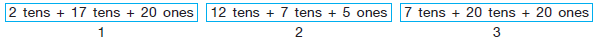# User Forum

Subject :IMO    Class : Class 2

Arrange the following from the greatest to the smallest.A1, 2, 3
B2, 3, 1
C3, 1, 2
D3, 2, 1

## Ans 1:

Class : Class 2
1 : 2 x 10 17 x 10 20 x 1 = 2102 : 12 x 10 7 x 10 5 x 1 = 1953 : 7 x 10 20 x 10 20 x 1 = 2903 > 1 > 2Close1 : 2 x 10 17 x 10 20 x 1 = 2102 : 12 x 10 7 x 10 5 x 1 = 1953 : 7 x 10 20 x 10 20 x 1 = 2903 > 1 > 2Close1 : 2 x 10 17 x 10 20 x 1 = 2102 : 12 x 10 7 x 10 5 x 1 = 1953 : 7 x 10 20 x 10 20 x 1 = 2903 > 1 > 2Close1 : 2 x 10 17 x 10 20 x 1 = 2102 : 12 x 10 7 x 10 5 x 1 = 1953 : 7 x 10 20 x 10 20 x 1 = 2903 > 1 > 2Close

Class : Class 1

(C): 1 : 20 + 170 + 20 = 210
2 : 120 + 70 + 5  = 195
3 : 70 + 200 + 20  = 290
As, 290>210>195

So, 3 > 1 > 2